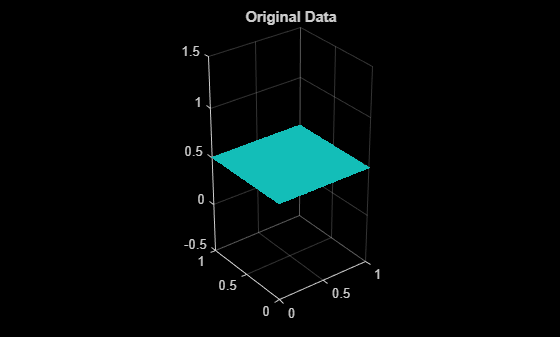# pcdenoise

Remove noise from 3-D point cloud

## Syntax

``ptCloudOut = pcdenoise(ptCloudIn)``
``````[ptCloudOut,inlierIndices,outlierIndices] = pcdenoise(ptCloudIn) ``````
``````[ptCloudOut,___] = pcdenoise(___Name=Value)``````

## Description

example

````ptCloudOut = pcdenoise(ptCloudIn)` returns a filtered point cloud that removes outliers.```
``````[ptCloudOut,inlierIndices,outlierIndices] = pcdenoise(ptCloudIn) ``` additionally returns the linear indices to the points that are identified as inliers and outliers.```
``````[ptCloudOut,___] = pcdenoise(___Name=Value)``` specifies a name-value argument in addition to any combination of arguments from previous syntaxes. For example, `Threshold=1.0` sets the outlier threshold to `1.0`.```

## Examples

collapse all

Create a plane point cloud.

```gv = 0:0.01:1; [X,Y] = meshgrid(gv,gv); ptCloud = pointCloud([X(:),Y(:),0.5*ones(numel(X),1)]); figure pcshow(ptCloud); title('Original Data');``````noise = rand(500, 3); ptCloudA = pointCloud([ptCloud.Location; noise]); figure pcshow(ptCloudA); title('Noisy Data');```Remove outliers.

```ptCloudB = pcdenoise(ptCloudA); figure; pcshow(ptCloudB); title('Denoised Data');```Load an organized point cloud from a saved MAT-file.

```ld = load('drivingLidarPoints.mat'); orgPtCloud = ld.ptCloud;```

Remove noise from the point cloud.

`orgPtCloudOut = pcdenoise(orgPtCloud,PreserveStructure=true);`

## Input Arguments

collapse all

Point cloud, specified as a `pointCloud` object.

### Name-Value Arguments

Specify optional pairs of arguments as `Name1=Value1,...,NameN=ValueN`, where `Name` is the argument name and `Value` is the corresponding value. Name-value arguments must appear after other arguments, but the order of the pairs does not matter.

Before R2021a, use commas to separate each name and value, and enclose `Name` in quotes.

Example: `Threshold=1.0` sets the threshold to `1.0`.

Number of nearest neighbor points, specified as the comma-separated pair consisting of '`NumNeighbors`' and a positive integer in pixels. The value is used to estimate the mean of the average distance to neighbors of all points. Decreasing this value makes the filter more sensitive to noise. Increasing this value increases the number of computations.

Data Types: `single` | `double`

Outlier threshold, specified as the comma-separated pair consisting of '`Threshold`' and a positive scalar. By default, the threshold is one standard deviation from the mean of the average distance to neighbors of all points. A point is considered to be an outlier if the average distance to its k-nearest neighbors is above the specified threshold.

Data Types: `single` | `double`

Preserve the organized structure of a point cloud as an M-by-N-by-3 location matrix, specified as `true` or `false`. The table describes the point cloud structure according to the value of `PreserveStructure`.

`PreserveStructure`The function returns
`true`

An organized, denoised, point cloud.

The Location property that describes the structure of the point cloud, contains an M-by-N-by-3 matrix.

Points that are not selected in the denoised point cloud are filled with `NaN`, and the corresponding color is set to ```[0 0 0]```.

To return an organized point cloud, the input must be an organized point cloud.

`false`

An unorganized, denoised, point cloud.

The Location property that describes the structure of the point cloud, contains an M-by-3 matrix.

## Output Arguments

collapse all

Filtered point cloud, returned as a `pointCloud` object.

Linear index of inlier points, returned as a 1-by-N vector.

Data Types: `uint32`

Linear index of outlier points, returned as a 1-by-N vector.

Data Types: `uint32`

 Rusu, R. B., Z. C. Marton, N. Blodow, M. Dolha, and M. Beetz. “Towards 3D Point Cloud Based Object Maps for Household Environments”. Robotics and Autonomous Systems Journal. 2008.